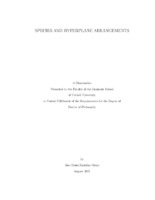## Species and Hyperplane Arrangements##### Abstract
This dissertation has two leading characters: Hopf monoids in the category of species and the Tits algebra of a real hyperplane arrangement. The relation between these two comes from the work of Aguiar and Mahajan (2013), who showed that a (co)commutative Hopf monoid gives rise to a family of (left)right-modules over the Tits algebra of the braid arrangement in all dimensions. One goal of this thesis is to explore the representation theory of the Tits algebra of arbitrary affine arrangements to extend what is known in the case of linear arrangements and to give an insight into some unanswered questions in the field of Hopf monoids. In the first part, we extend the study of characteristic elements of a hyperplane arrangement from the linear to the affine case. We present the basic properties of these elements and apply them to derive numerous results about the characteristic polynomial of an arrangement, from Zaslavsky's formulas to more recent results of Kung and of Klivans and Swartz. We construct several examples of characteristic elements, including one in terms of intrinsic volumes of faces of the arrangement. In the second part, we study deformations $\arr$ of a linear arrangement $\arr_0$ and endow the Tits algebra of $\arr$ with a bimodule structure over the algebra of $\arr_0$. The left module structure sheds some light on the study of exponential sequences of arrangements, in the sense of Stanley. In particular, we construct the Hopf monoid of faces associated with such a sequence and use characteristic elements to deduce formulas for certain bivariate polynomial invariants of these arrangements. In the third part, we endow the polytope subalgebra of deformations of a zonotope with the structure of a module over the Tits algebra of the corresponding hyperplane arrangement. We study algebraic invariants of this module and find relations between statistics on (signed) permutations and the module structure in the case of (type B) generalized permutahedra. In type B, the module structure surprisingly reveals that any family of generators (via signed Minkowski sums) for generalized permutahedra of type B will contain at least $2^{d-1}$ full-dimensional polytopes. We find a generating family of simplices attaining this minimum. Finally, we prove that the relations defining the polytope algebra are compatible with the Hopf monoid structure of generalized permutahedra, and explain the relationship between the antipode formula of this Hopf monoid and inversion in the polytope algebra. In the last chapter, we introduce a novel definition of type B Hopf monoids. Unlike standard Hopf monoids and the Hopf monoids in $\cH$-species of Bergeron and Choquette (2009), our notion involves a pair of species (one of type A with an involution and one of type B) and a (co)module structure of one over the other. This closer represents the algebraic structure that arises from the Tits algebra of the type B Coxeter arrangement. We study some general constructions like the substitution product of type B objects and the free (commutative) monoid over a positive type B object. We conclude by endowing the type B object of generalized permutahedra and the type B object of symplectic matroids with the structure of a type B Hopf monoid.
199 pages
2021-08
##### Keywords
Generalized permutahedra; Hopf monoids; Hyperplane arrangements; Species
Aguiar, Marcelo
##### Committee Member
Swartz, Ed
Stillman, Michael
Mathematics
##### Degree Name
Ph. D., Mathematics
##### Degree Level
Doctor of Philosophy